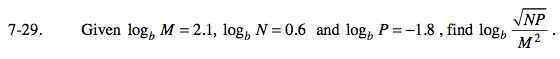### Home > PC > Chapter 7 > Lesson 7.1.2 > Problem7-29

7-29.Break this up into many logs. Then substitute the given values.

$\log_{b}\sqrt{N}+\log_{b}\sqrt{P}-\log_{b}M^{2}$

$\left ( \frac{1}{2} \right )\text{log}_{b}N+\left ( \frac{1}{2} \right )\text{log}_{b}P-2\text{log}_{b}M$

Substitute the known values in the expression above.